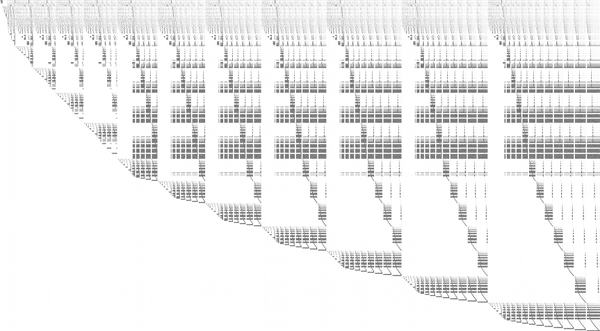## 10 Search Results

### Givaro

In the joint CNRS-INRIA / INPG-UJF project APACHE, Givaro is a C++ library for arithmetic and algebraic computations. Its main features are implementations of the basic arithmetic of many mathematical entities: Primes fields, Extensions Fields, Finite Fields, Finite Rings, Polynomials, Algebraic numbers, Arbitrary precision integers and rationals (C++ wrappers over gmp) It also provides data-structures and templated classes for the manipulation of basic algebraic objects, such as vectors, matrices (dense, sparse, structured), univariate polynomials (and therefore recursive multivariate). It contains different program modules and is fully compatible with the LinBox linear algebra library and the Athapascan environment, which permits parallel programming.

### IML - Integer Matrix Library

IML is a free library of C source code which implements algorithms for computing exact solutions to dense systems of linear equations over the integers. IML is designed to be used with the ATLAS/BLAS library and GMP bignum library. Written in portable C, IML can be used on both 32-bit and 64-bit machines. It can be called from C++.

### LAPACK

LAPACK is written in Fortran77 and provides routines for solving systems of simultaneous linear equations, least-squares solutions of linear systems of equations, eigenvalue problems, and singular value problems. The associated matrix factorizations (LU, Cholesky, QR, SVD, Schur, generalized Schur) are also provided, as are related computations such as reordering of the Schur factorizations and estimating condition numbers. Dense and banded matrices are handled, but not general sparse matrices. In all areas, similar functionality is provided for real and complex matrices, in both single and double precision.

### LinBox

LinBox is a C++ template library for exact, high-performance linear algebra computation with dense, sparse, and structured matrices over the integers and over finite fields. LinBox has the following top-level functions: solve linear system, matrix rank, determinant, minimal polynomial, characteristic polynomial, Smith normal form and trace. A good collection of finite field and ring implementations is provided, for use with numerous black box matrix storage schemes.

### M4RI

M4RI is a library for fast arithmetic with dense matrices over F2.

More information### Mathematica

Mathematica seamlessly integrates a numeric and symbolic computational engine, graphics system, programming language, documentation system, and advanced connectivity to other applications.

### Risa/Asir

Risa/Asir is a general computer algebra system and also a tool for various computation in mathematics and engineering. The development of Risa/Asir started in 1989 at FUJITSU. Binaries have been freely available since 1994 and now the source code is also free. Currently Kobe distribution is the most active branch of its development. We characterize Risa/Asir as follows: (1) An environment for large scale and efficient polynomial computation. (2) A platform for parallel and distributed computation based on OpenXM protocols.

### Sage

SAGE is a framework for number theory, algebra, and geometry computation. It is open source and freely available under the terms of the GNU General Public License (GPL). SAGE is a Python library with a customized interpreter. It is written in Python, C++, and C (via Pyrex). Python (http://www.python.org) is an open source object-oriented interpreted language, with a large number of libraries, e.g., for numerical analysis, which are available to users of SAGE. Python can also be accessed in library mode from C/C++ programs. SAGE provides an interface to several important open source libraries, including Cremona’s MWRANK library for computing with elliptic curves, the PARI library (pari.math.u-bordeaux.fr) for number theory, Shoup’s number theory library NTL (http://www.shoup.net/ntl/), SINGULAR (http://www.singular.uni-kl.de) for commutative algebra, GAP (http://www.gap-system.org) for group theory and combinatorics, and maxima (http://maxima.sourceforge.net) for symbolic computation and calculus.

More information### SuperLU

SuperLU is a general purpose library for the direct solution of large, sparse, nonsymmetric systems of linear equations on high performance machines. The library is written in C and is callable from either C or Fortran. The library routines will perform an LU decomposition with partial pivoting and triangular system solves through forward and back substitution. The LU factorization routines can handle non-square matrices but the triangular solves are performed only for square matrices. The matrix columns may be preordered (before factorization) either through library or user supplied routines. This preordering for sparsity is completely separate from the factorization. Working precision iterative refinement subroutines are provided for improved backward stability. Routines are also provided to equilibrate the system, estimate the condition number, calculate the relative backward error, and estimate error bounds for the refined solutions.